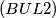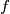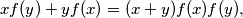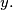### IMO Shortlist 1969 problem 8

Kvaliteta:
Avg: 3.0
Težina:
Avg: 4.0$(BUL 2)$ Find all functions$f$ defined for all$x$ that satisfy the condition$xf(y) + yf(x) = (x + y)f(x)f(y),$ for all$x$ and$y.$ Prove that exactly two of them are continuous.
Source: Međunarodna matematička olimpijada, shortlist 1969